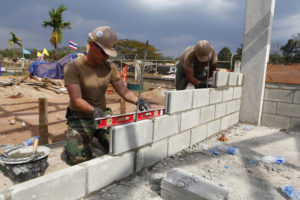## Why understand how equations and inequalities help in the real world?U.S. Navy Seabees help build a classroom for a school in Thailand.

You are in charge of planning and purchasing materials for a new school to be built overseas in a small village. The plans for the foundation indicate that it must be rectangular with a length of exactly 100 feet greater than the width. However, the total area must be 12,000 sq. ft. How long and how wide should the foundation be?

First we have to model the problem using mathematics. Let $x$ represent the width (shorter side). Then the length must be $x+100$. Using the area formula for a rectangle, we find:

$Area=\left(length\right)\times\left(width\right)=\left(x+100\right)\left(x\right)=x^2+100x$

Now since the total area must be equal to 12,000, we can write:

$x^2+100x=12,000$

or equivalently,

$x^2+100x-12,000=0$

This is an equation, which is a mathematical statement consisting of two expressions set equal to one another. In order to find out the proper length and width of the floor, we would have to solve the equation for the variable x using techniques that you will soon discover.

Suppose the walls of the school will be built from concrete blocks costing $1.20 per block (which includes tax) plus a flat fee of$100 for hauling the blocks to the site. Having only a limited budget, you must be very careful to order only what you can afford. Suppose the school requires at least 1800 concrete blocks (but having more blocks around for future projects would not be a bad idea). Your budgeted amount for concrete blocks is $2,400. What is the maximum number of concrete blocks that you can purchase? Do you have enough money to buy at least the minimum number of concrete blocks required for the project? To answer the question above, we must be familiar with inequalities. An inequality is a statement involving relations like “less than,” “more than,” “at least,” or “at most.” For example, if the number of blocks to be purchased is n, then there are two inequalities implied by the story. $n\ge1800$ and $(1.20)n+100\le2400$ The first inequality expresses the fact that at least 1800 blocks are required. The second inequality states that the total cost of the blocks, together with the hauling fee, cannot exceed the budgeted amount of$2,400.

In this module, you will learn how to analyze this situation as well as many others that involve building and solving equations and inequalities. We will revisit our construction site again at the end of the module.